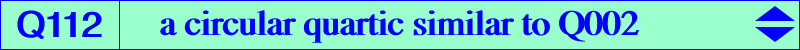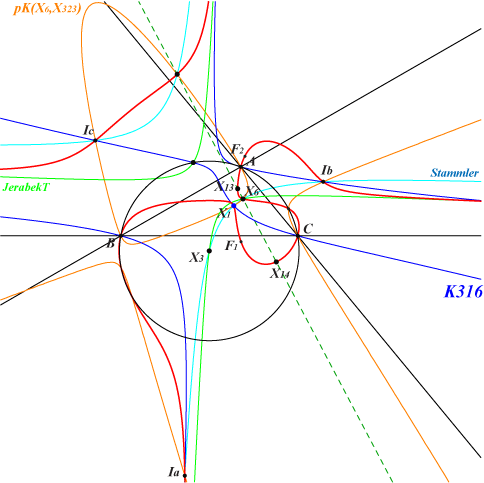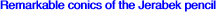too complicated to be written here. Click on the link to download a text file.X(1), X(6), X(13), X(14), X(399), X(2574), X(2575) excenters foci of the K-ellipse (inellipse with center K when the triangle ABC is acute angle) points of pK(X6, X323) on (O)Let L be a line passing through X(3) and M a variable point on L. Denote by J(M) the rectangular hyperbola passing through M, X(3), X(6), X(2574), X(2575), hence homothetic to the Jerabek hyperbola. J(M) is a member of the Jerabek pencil, see below. Denote by K(M) the isogonal pivotal cubic with pivot M i.e. pK(X6, M). J(M) and K(M) meet at M and five other points which generally lie on a circular quartic Q(L), together with the line L. Trivial cases : • when L is parallel to one asymptote of the Jerabek hyperbola, J(M) splits into L and its perpendicular at X(6). • when L is the Brocard axis, J(M) splits into this axis and the line at infinity. In both cases, J(M) is independent of M and Q(L) is not a quartic but J(M) itself. This is excluded in the sequel. Properties : When L rotates about X(3), Q(L) contains 16 fixed points therefore these quartics are in a same pencil. These points are : • X(2574), X(2575), two circular points at infinity, • A, B, C, the four in/excenters, X(6), the four foci of the inconic with center X(6) sometimes called ellipse-K when ABC is acutangle. Furthermore, Q(L) meets the circumcircle (O) again at the same (not always real) points as pK(X6, Z) where Z is a point on the line X(2)X(6), the barycentric product of X(99) and the infinite point of a perpendicular to L. Examples : • when L is the Euler line, Q(L) is Q002, Z = X(2), pK(X6, Z) is K002 and Q(L) meets (O) at the vertices of the Thomson triangle. • when L is the line through X(110), Q(L) is Q112, Z = X(323).Recall that the members of the Jerabek pencil are the rectangular hyperbolas passing through X(3), X(6), X(2574), X(2575). Their centers Ω lie on the line containing X(2), X(98), X(110), X(114), X(125), X(147), X(182), X(184), X(287), X(542), X(1352), X(1899), X(1976), X(2001), X(3047), etc. • Ω = X(542), union of the line at infinity and the Brocard axis. • Ω = X(125), the Jerabek hyperbola itself, the only circum-conic. • Ω = X(110), the Stammler hyperbola, the only diagonal conic. • Ω = X(5642), the Jerabek hyperbola of the Thomson triangle. • Ω = X(5972), the complement of the Jerabek hyperbola, the bicevian conic C(X2, X110). These hyperbolas are the polar conics of X(3) in a cubic of the Euler pencil or the polar conic in K003 of a point on the Euler line. Further details in the page Q002.